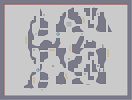### Eternal | PitfallHover over the thumbnail for a full-size version.

Author Arsenicc author:arsenicc beatable drones jumps nv2 unrated 2017-10-13 2017-10-14 2 more votes required for a rating. \$Eternal | Pitfall#Arsenicc#jumps#000000000000000000000000000000000000000000000000000000000000000000000000000000000000000000000000000000000000000000000000000000000000000C000000GOK1111LOH000GO1100000OE00FQ0B00BO00E00F00000000GO00NH0000000GO000FQN11N1031P11PQI11N1000000B1NE011P1E000B1NM00000000000B1000000000000000000000G10000000000000000G110N1M11H000G1100000G111100001111H011100000QI0FQ0000QI0FQ00FQ0000C1D0000000000C1D0000000Q11H?100011@G11QH?100000Q11N10GK11P11Q01N1000000FQ0201MI50QI00Q0200001O000000O10000O10000000QQ0Q1000N101Q0QQQ10000011Q0Q000B10Q0Q110Q00000I0OOKD0000CLOO0FOKD00000111110000111110111000000FQQQ0000QQQI00QQQ00000000FJ000000000000000000000000000000000000000000000000000000000000000000000000000000000000000000000000000000000000|5^618,276!12^768,24!12^768,36!12^768,30!12^768,42!12^768,42!12^768,48!12^768,54!12^768,66!12^768,60!12^768,72!12^768,72!12^768,84!12^768,78!12^768,90!12^768,90!12^768,96!12^768,102!12^768,114!12^768,108!12^768,120!12^768,120!12^768,132!12^768,126!12^768,138!12^768,138!12^768,144!12^768,150!12^768,162!12^768,156!12^768,168!12^768,120!12^768,132!12^768,126!12^768,138!12^768,138!12^768,144!12^768,150!12^768,162!12^768,156!12^768,168!12^768,144!12^768,156!12^768,150!12^768,162!12^768,162!12^768,168!12^768,174!12^768,186!12^768,180!12^768,192!12^768,192!12^768,204!12^768,198!12^768,210!12^768,210!12^768,216!12^768,222!12^768,234!12^768,228!12^768,240!12^768,216!12^768,228!12^768,222!12^768,234!12^768,234!12^768,240!12^768,246!12^768,258!12^768,252!12^768,264!12^768,264!12^768,276!12^768,270!12^768,282!12^768,282!12^768,288!12^768,294!12^768,306!12^768,300!12^768,312!12^768,288!12^768,300!12^768,294!12^768,306!12^768,306!12^768,312!12^768,318!12^768,330!12^768,324!12^768,336!12^768,288!12^768,300!12^768,294!12^768,306!12^768,306!12^768,312!12^768,318!12^768,330!12^768,324!12^768,336!12^768,336!12^768,348!12^768,342!12^768,354!12^768,354!12^768,360!12^768,366!12^768,378!12^768,372!12^768,384!12^768,384!12^768,396!12^768,390!12^768,402!12^768,402!12^768,408!12^768,414!12^768,426!12^768,420!12^768,432!12^768,408!12^768,420!12^768,414!12^768,426!12^768,426!12^768,432!12^768,438!12^768,450!12^768,444!12^768,456!12^768,432!12^768,444!12^768,438!12^768,450!12^768,450!12^768,456!12^768,462!12^768,474!12^768,468!12^768,480!12^768,456!12^768,468!12^768,462!12^768,474!12^768,474!12^768,480!12^768,486!12^768,498!12^768,492!12^768,504!12^768,480!12^768,492!12^768,486!12^768,498!12^768,498!12^768,504!12^768,510!12^768,522!12^768,516!12^768,528!12^768,528!12^768,540!12^768,534!12^768,546!12^768,546!12^768,552!12^768,558!12^768,570!12^768,564!12^768,576!12^24,24!12^24,36!12^24,30!12^24,42!12^24,42!12^24,48!12^24,54!12^24,66!12^24,60!12^24,72!12^24,72!12^24,84!12^24,78!12^24,90!12^24,90!12^24,96!12^24,102!12^24,114!12^24,108!12^24,120!12^24,120!12^24,132!12^24,126!12^24,138!12^24,138!12^24,144!12^24,150!12^24,162!12^24,156!12^24,168!12^24,120!12^24,132!12^24,126!12^24,138!12^24,138!12^24,144!12^24,150!12^24,162!12^24,156!12^24,168!12^24,144!12^24,156!12^24,150!12^24,162!12^24,162!12^24,168!12^24,174!12^24,186!12^24,180!12^24,192!12^24,192!12^24,204!12^24,198!12^24,210!12^24,210!12^24,216!12^24,222!12^24,234!12^24,228!12^24,240!12^24,216!12^24,228!12^24,222!12^24,234!12^24,234!12^24,240!12^24,246!12^24,258!12^24,252!12^24,264!12^24,264!12^24,276!12^24,270!12^24,282!12^24,282!12^24,288!12^24,294!12^24,306!12^24,300!12^24,312!12^24,288!12^24,300!12^24,294!12^24,306!12^24,306!12^24,312!12^24,318!12^24,330!12^24,324!12^24,336!12^24,288!12^24,300!12^24,294!12^24,306!12^24,306!12^24,312!12^24,318!12^24,330!12^24,324!12^24,336!12^24,336!12^24,348!12^24,342!12^24,354!12^24,354!12^24,360!12^24,366!12^24,378!12^24,372!12^24,384!12^24,384!12^24,396!12^24,390!12^24,402!12^24,402!12^24,408!12^24,414!12^24,426!12^24,420!12^24,432!12^24,408!12^24,420!12^24,414!12^24,426!12^24,426!12^24,432!12^24,438!12^24,450!12^24,444!12^24,456!12^24,432!12^24,444!12^24,438!12^24,450!12^24,450!12^24,456!12^24,462!12^24,474!12^24,468!12^24,480!12^24,456!12^24,468!12^24,462!12^24,474!12^24,474!12^24,480!12^24,486!12^24,498!12^24,492!12^24,504!12^24,480!12^24,492!12^24,486!12^24,498!12^24,498!12^24,504!12^24,510!12^24,522!12^24,516!12^24,528!12^24,528!12^24,540!12^24,534!12^24,546!12^24,546!12^24,552!12^24,558!12^24,570!12^24,564!12^24,576!12^576,24!12^564,24!12^570,24!12^558,24!12^558,24!12^552,24!12^546,24!12^534,24!12^540,24!12^528,24!12^528,24!12^516,24!12^522,24!12^510,24!12^510,24!12^504,24!12^498,24!12^486,24!12^492,24!12^480,24!12^480,24!12^468,24!12^474,24!12^462,24!12^462,24!12^456,24!12^450,24!12^438,24!12^444,24!12^432,24!12^480,24!12^468,24!12^474,24!12^462,24!12^462,24!12^456,24!12^450,24!12^438,24!12^444,24!12^432,24!12^456,24!12^444,24!12^450,24!12^438,24!12^438,24!12^432,24!12^426,24!12^414,24!12^420,24!12^408,24!12^408,24!12^396,24!12^402,24!12^390,24!12^390,24!12^384,24!12^378,24!12^366,24!12^372,24!12^360,24!12^384,24!12^372,24!12^378,24!12^366,24!12^366,24!12^360,24!12^354,24!12^342,24!12^348,24!12^336,24!12^336,24!12^324,24!12^330,24!12^318,24!12^318,24!12^312,24!12^306,24!12^294,24!12^300,24!12^288,24!12^312,24!12^300,24!12^306,24!12^294,24!12^294,24!12^288,24!12^282,24!12^270,24!12^276,24!12^264,24!12^312,24!12^300,24!12^306,24!12^294,24!12^294,24!12^288,24!12^282,24!12^270,24!12^276,24!12^264,24!12^264,24!12^252,24!12^258,24!12^246,24!12^246,24!12^240,24!12^234,24!12^222,24!12^228,24!12^216,24!12^216,24!12^204,24!12^210,24!12^198,24!12^198,24!12^192,24!12^186,24!12^174,24!12^180,24!12^168,24!12^192,24!12^180,24!12^186,24!12^174,24!12^174,24!12^168,24!12^162,24!12^150,24!12^156,24!12^144,24!12^168,24!12^156,24!12^162,24!12^150,24!12^150,24!12^144,24!12^138,24!12^126,24!12^132,24!12^120,24!12^144,24!12^132,24!12^138,24!12^126,24!12^126,24!12^120,24!12^114,24!12^102,24!12^108,24!12^96,24!12^120,24!12^108,24!12^114,24!12^102,24!12^102,24!12^96,24!12^90,24!12^78,24!12^84,24!12^72,24!12^72,24!12^60,24!12^66,24!12^54,24!12^54,24!12^48,24!12^42,24!12^30,24!12^36,24!12^24,24!12^768,24!12^756,24!12^762,24!12^750,24!12^750,24!12^744,24!12^738,24!12^726,24!12^732,24!12^720,24!12^720,24!12^708,24!12^714,24!12^702,24!12^702,24!12^696,24!12^690,24!12^678,24!12^684,24!12^672,24!12^672,24!12^660,24!12^666,24!12^654,24!12^654,24!12^648,24!12^642,24!12^630,24!12^636,24!12^624,24!12^672,24!12^660,24!12^666,24!12^654,24!12^654,24!12^648,24!12^642,24!12^630,24!12^636,24!12^624,24!12^648,24!12^636,24!12^642,24!12^630,24!12^630,24!12^624,24!12^618,24!12^606,24!12^612,24!12^600,24!12^600,24!12^588,24!12^594,24!12^582,24!12^582,24!12^576,24!12^570,24!12^558,24!12^564,24!12^552,24!12^576,24!12^564,24!12^570,24!12^558,24!12^558,24!12^552,24!12^546,24!12^534,24!12^540,24!12^528,24!12^528,24!12^516,24!12^522,24!12^510,24!12^510,24!12^504,24!12^498,24!12^486,24!12^492,24!12^480,24!12^504,24!12^492,24!12^498,24!12^486,24!12^486,24!12^480,24!12^474,24!12^462,24!12^468,24!12^456,24!12^504,24!12^492,24!12^498,24!12^486,24!12^486,24!12^480,24!12^474,24!12^462,24!12^468,24!12^456,24!12^456,24!12^444,24!12^450,24!12^438,24!12^438,24!12^432,24!12^426,24!12^414,24!12^420,24!12^408,24!12^408,24!12^396,24!12^402,24!12^390,24!12^390,24!12^384,24!12^378,24!12^366,24!12^372,24!12^360,24!12^384,24!12^372,24!12^378,24!12^366,24!12^366,24!12^360,24!12^354,24!12^342,24!12^348,24!12^336,24!12^360,24!12^348,24!12^354,24!12^342,24!12^342,24!12^336,24!12^330,24!12^318,24!12^324,24!12^312,24!12^336,24!12^324,24!12^330,24!12^318,24!12^318,24!12^312,24!12^306,24!12^294,24!12^300,24!12^288,24!12^312,24!12^300,24!12^306,24!12^294,24!12^294,24!12^288,24!12^282,24!12^270,24!12^276,24!12^264,24!12^264,24!12^252,24!12^258,24!12^246,24!12^246,24!12^240,24!12^234,24!12^222,24!12^228,24!12^216,24!12^24,576!12^36,576!12^30,576!12^42,576!12^42,576!12^48,576!12^54,576!12^66,576!12^60,576!12^72,576!12^72,576!12^84,576!12^78,576!12^90,576!12^90,576!12^96,576!12^102,576!12^114,576!12^108,576!12^120,576!12^120,576!12^132,576!12^126,576!12^138,576!12^138,576!12^144,576!12^150,576!12^162,576!12^156,576!12^168,576!12^120,576!12^132,576!12^126,576!12^138,576!12^138,576!12^144,576!12^150,576!12^162,576!12^156,576!12^168,576!12^144,576!12^156,576!12^150,576!12^162,576!12^162,576!12^168,576!12^174,576!12^186,576!12^180,576!12^192,576!12^192,576!12^204,576!12^198,576!12^210,576!12^210,576!12^216,576!12^222,576!12^234,576!12^228,576!12^240,576!12^216,576!12^228,576!12^222,576!12^234,576!12^234,576!12^240,576!12^246,576!12^258,576!12^252,576!12^264,576!12^264,576!12^276,576!12^270,576!12^282,576!12^282,576!12^288,576!12^294,576!12^306,576!12^300,576!12^312,576!12^288,576!12^300,576!12^294,576!12^306,576!12^306,576!12^312,576!12^318,576!12^330,576!12^324,576!12^336,576!12^288,576!12^300,576!12^294,576!12^306,576!12^306,576!12^312,576!12^318,576!12^330,576!12^324,576!12^336,576!12^336,576!12^348,576!12^342,576!12^354,576!12^354,576!12^360,576!12^366,576!12^378,576!12^372,576!12^384,576!12^384,576!12^396,576!12^390,576!12^402,576!12^402,576!12^408,576!12^414,576!12^426,576!12^420,576!12^432,576!12^408,576!12^420,576!12^414,576!12^426,576!12^426,576!12^432,576!12^438,576!12^450,576!12^444,576!12^456,576!12^432,576!12^444,576!12^438,576!12^450,576!12^450,576!12^456,576!12^462,576!12^474,576!12^468,576!12^480,576!12^456,576!12^468,576!12^462,576!12^474,576!12^474,576!12^480,576!12^486,576!12^498,576!12^492,576!12^504,576!12^480,576!12^492,576!12^486,576!12^498,576!12^498,576!12^504,576!12^510,576!12^522,576!12^516,576!12^528,576!12^528,576!12^540,576!12^534,576!12^546,576!12^546,576!12^552,576!12^558,576!12^570,576!12^564,576!12^576,576!12^216,576!12^228,576!12^222,576!12^234,576!12^234,576!12^240,576!12^246,576!12^258,576!12^252,576!12^264,576!12^264,576!12^276,576!12^270,576!12^282,576!12^282,576!12^288,576!12^294,576!12^306,576!12^300,576!12^312,576!12^312,576!12^324,576!12^318,576!12^330,576!12^330,576!12^336,576!12^342,576!12^354,576!12^348,576!12^360,576!12^312,576!12^324,576!12^318,576!12^330,576!12^330,576!12^336,576!12^342,576!12^354,576!12^348,576!12^360,576!12^336,576!12^348,576!12^342,576!12^354,576!12^354,576!12^360,576!12^366,576!12^378,576!12^372,576!12^384,576!12^384,576!12^396,576!12^390,576!12^402,576!12^402,576!12^408,576!12^414,576!12^426,576!12^420,576!12^432,576!12^408,576!12^420,576!12^414,576!12^426,576!12^426,576!12^432,576!12^438,576!12^450,576!12^444,576!12^456,576!12^456,576!12^468,576!12^462,576!12^474,576!12^474,576!12^480,576!12^486,576!12^498,576!12^492,576!12^504,576!12^480,576!12^492,576!12^486,576!12^498,576!12^498,576!12^504,576!12^510,576!12^522,576!12^516,576!12^528,576!12^480,576!12^492,576!12^486,576!12^498,576!12^498,576!12^504,576!12^510,576!12^522,576!12^516,576!12^528,576!12^528,576!12^540,576!12^534,576!12^546,576!12^546,576!12^552,576!12^558,576!12^570,576!12^564,576!12^576,576!12^576,576!12^588,576!12^582,576!12^594,576!12^594,576!12^600,576!12^606,576!12^618,576!12^612,576!12^624,576!12^600,576!12^612,576!12^606,576!12^618,576!12^618,576!12^624,576!12^630,576!12^642,576!12^636,576!12^648,576!12^624,576!12^636,576!12^630,576!12^642,576!12^642,576!12^648,576!12^654,576!12^666,576!12^660,576!12^672,576!12^648,576!12^660,576!12^654,576!12^666,576!12^666,576!12^672,576!12^678,576!12^690,576!12^684,576!12^696,576!12^672,576!12^684,576!12^678,576!12^690,576!12^690,576!12^696,576!12^702,576!12^714,576!12^708,576!12^720,576!12^720,576!12^732,576!12^726,576!12^738,576!12^738,576!12^744,576!12^750,576!12^762,576!12^756,576!12^768,576!12^360,258!12^444,192!12^468,84!12^378,84!12^366,504!12^540,312!12^486,372!12^276,312!12^252,180!12^186,132!12^588,186!12^564,48!12^438,48!12^450,444!12^288,468!12^204,372!12^186,528!0^300,300!0^312,300!0^324,300!0^420,300!0^420,288!0^420,276!0^648,336!0^648,324!0^648,312!0^648,300!0^156,258!0^156,246!0^156,234!0^156,222!0^204,492!0^204,480!0^204,468!0^396,444!0^396,456!0^402,468!0^498,108!0^504,120!0^504,132!11^414,144,588,396!9^414,354,0,0,25,17,1,0,0!9^588,84,0,0,25,17,1,0,0!9^204,300,0,0,24,17,1,0,0!9^264,90,0,0,24,17,1,0,0!9^510,444,0,0,25,17,1,0,0!9^492,204,1,1,26,22,0,0,0!9^216,204,1,1,9,22,0,0,0!9^216,204,1,1,10,22,0,0,0!9^216,204,1,1,11,22,0,0,0!9^252,540,0,1,5,15,0,0,0!7^528,516,3!6^348,204,0,0,0,0!6^228,420,0,0,0,3!3^318,396# tiles from deep97's pitfall (301799 on nv2) inspired by lusprs First map posted on NUMA. "I don't believe in pitfalls. I believe in taking risks and not doing the same thing twice." - Guy Laliberte

Pages: (0)

### Hey there!

Glad to finally see you on NUMA! I'm sure the people here are going to love you!

As for the map, I'll give it a go when I get a chance, looks great!

### Thanks Hindi

Yeah I noticed. When I tested it I was focused on the drone movement because I thought it was different than nv2's drone movement. I will fix for sure ;)

### Welcome, Arsenicc!

Firstly, welcome to NUMA! I'm kind of new myself, but I'm loving it already.